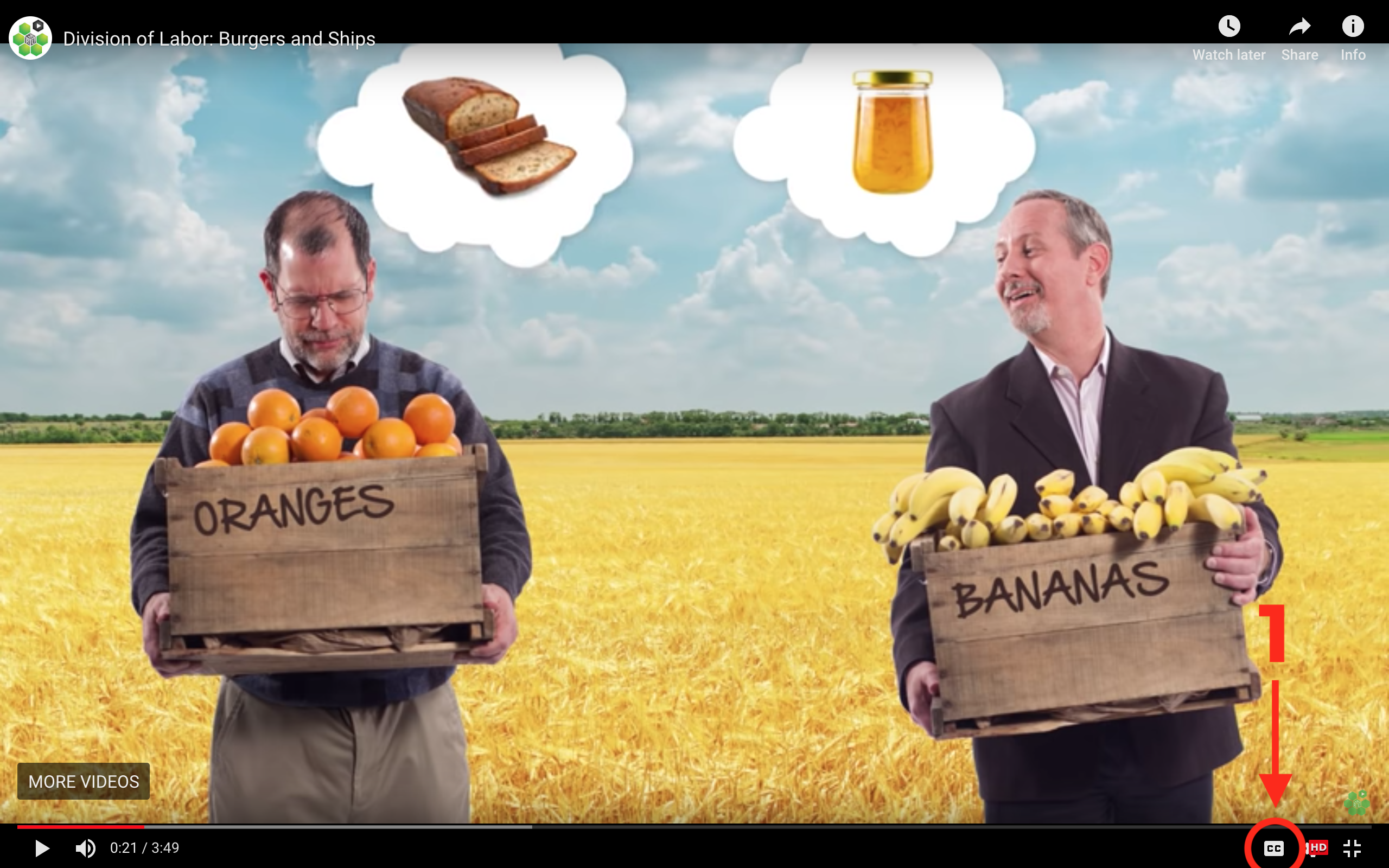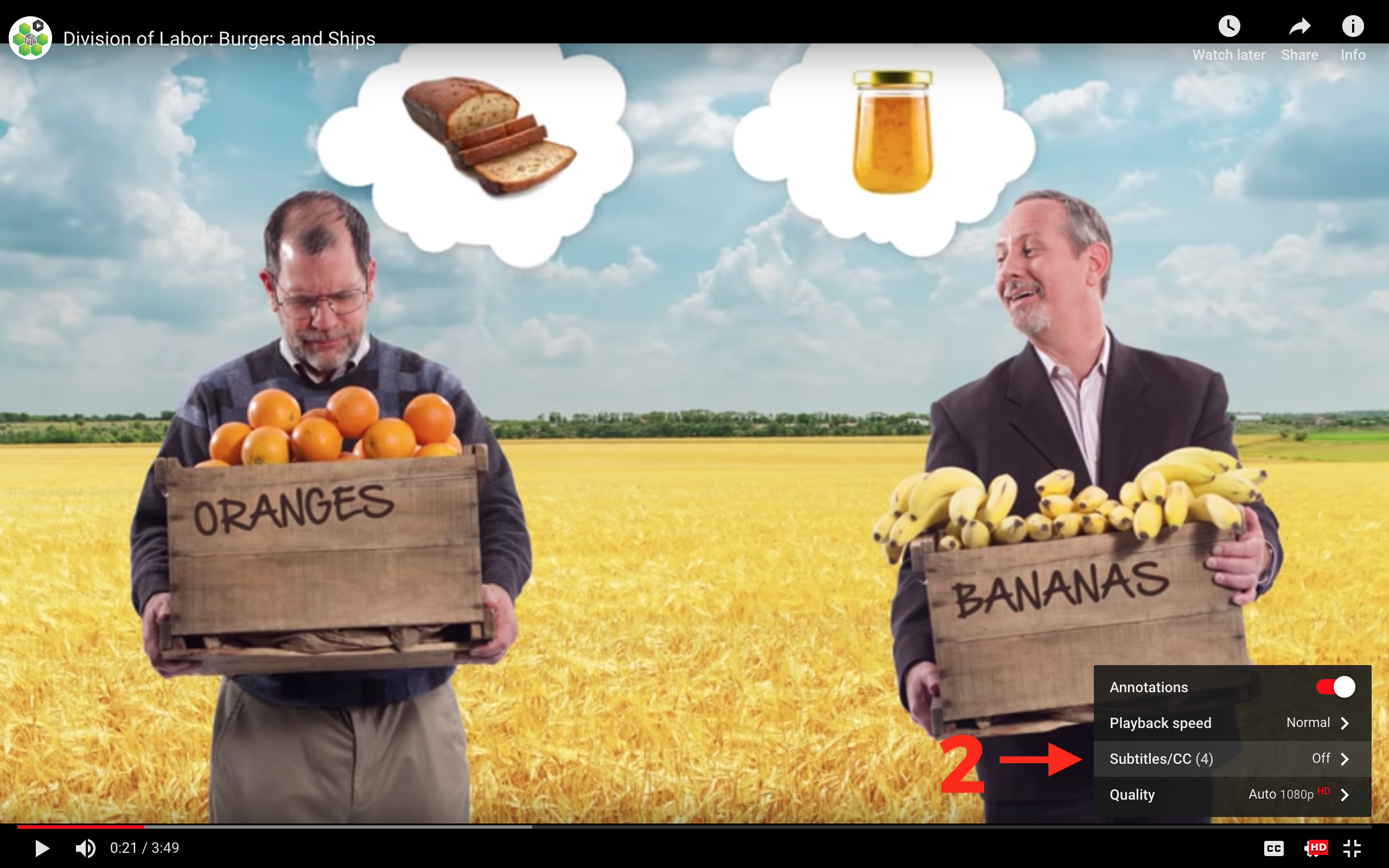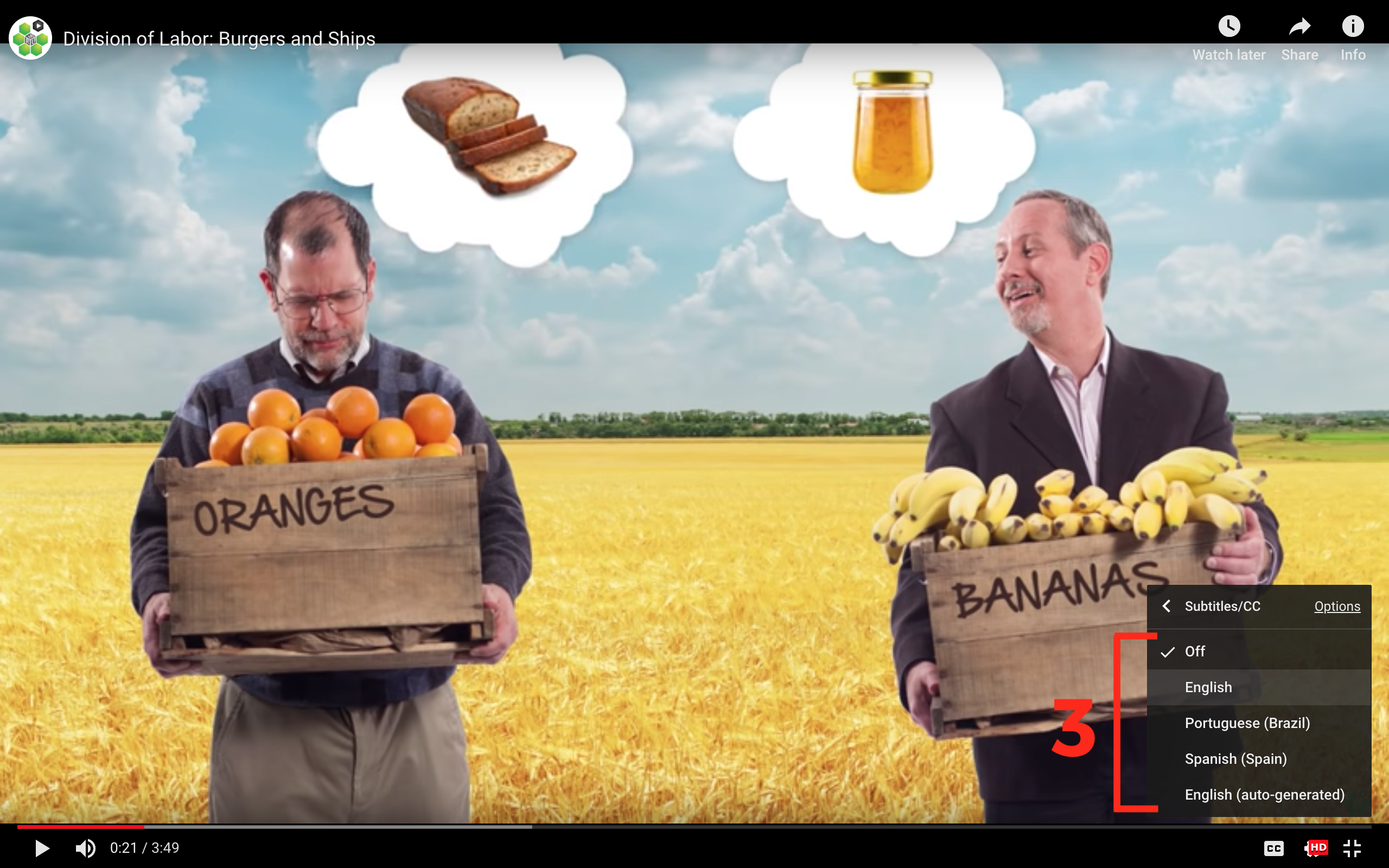## Minimization of Total Industry Costs of Production

This section connects several ideas covered in previous videos about the price system and profit maximization. In this video, we begin to understand two basic functions of the Invisible Hand. In competitive markets, the market price (with the help of the Invisible Hand) balances production across firms so that total industry costs are minimized. Competitive markets also connect different industries. By balancing production, the Invisible Hand of the market ensures that the total value of production is maximized across different industries. We’ll use the example of minimizing total costs of corn production, and demonstrate our findings through several charts.

## Transcript

In this chapter, we return to the invisible hand. We're going to show some remarkable properties of competitive markets, properties that are a product of human action but not of human design. That is, these properties have neither been designed nor intended, nor perhaps even understood by market participants. And yet through the process of the invisible hand, a spontaneous order evolves in which these desirable properties are an outcome. Let's take a look.

So for context, recall that in earlier chapters we learned that markets connect and coordinate actions all over the world. Think about the rose and the coordination of actions which was necessary to deliver the fresh rose to your door on Valentine's Day. We also learned that a price is a signal wrapped up in an incentive. That is, prices signal in what uses resources have the highest value and they provide an incentive to move resources to those high-valued uses. We also learned that firms maximize profits by doing two things. First, by producing at the quantity where price is equal to marginal cost. And second, by entering an industry when there's profits, when price is greater than average cost, and by exiting an industry when there are losses, when price is less than average cost.

What this chapter is all about is connecting these ideas, bringing these ideas together. We're going to show that competitive markets have two remarkable invisible hand properties. First, competitive markets balance production across firms in an industry, so that total industry costs are minimized for any given quantity of production. Second, entry and exit decisions balance production across different industries so that the total value of production is maximized. And we'll explain each of these in turn.

To show how the invisible hand minimizes total industry cost, we're going to start with what looks to be a quite different problem. Suppose that you owned two farms and you want to produce 200 bushels of corn at the lowest possible cost. How do you do it? Well by looking at these two marginal cost curves, you might reason that since the cost of producing any quantity of corn is lower on Farm Two than on Farm One, then maybe the best thing to do is to produce all 200 units on Farm Two.

I'm going to show you that that's wrong. Now, let's remember that we could read the cost of producing the Nth unit of corn as the height of the marginal cost curve for that unit. So here's the cost of producing the 200th unit of corn. Now imagine that you produced all 200 units from Farm Two. Let's now show a lower cost way of producing 200 units. For example, suppose you were to produce 25 fewer units on Farm Two. Your cost will then fall by area A. Now of course now you're producing 25 units less, so in order to make up for that decrease in production, you've got to produce 25 more units from Farm One. Notice that in order to produce those 25 units on Farm One, your costs go up by area B.

Now here's the key point -- area A is bigger than area B. In other words by switching cost from the high marginal cost farm to the low marginal cost farm, you have decreased your costs by more than you have increased your costs. In fact you've created a savings of area C, the difference. Now if you follow through this logic, it implies that whenever the marginal cost on one farm is higher than the marginal cost on the other farm, you can save money, you can save resources, by transferring production from where the marginal cost is high to where the marginal cost is low.

Now what does that mean if you want to minimize the total cost of production? The logic we just gave implies that if you want to minimize the total cost of production, you should balance your production across the two farms so that the marginal costs on the two farms are equal. In this case a 160 units from Farm Two and 40 units from Farm One. Again, just think about if that were not the case. If the marginal cost of production on Farm Two were higher than on Farm One, then you could always reduce your cost by producing less on Farm Two and more on Farm One. But of course the reverse is also true. If the marginal cost on Farm One were higher than on Farm Two, you would want to produce less on Farm One and more on Farm Two. So the way to minimize your total cost of production is to produce such that the marginal costs of production are equal on your two farms.

Now let's consider a much more difficult problem. Suppose we have Pat's farm located on the West Coast and Alex's farm thousands of miles away on the East Coast. And let us suppose that no one knows the marginal cost on both of these farms. Now the problem looks almost impossible. How could we possibly allocate production across these two farms to minimize total costs when no one knows the marginal cost on both of these farms? Clearly a central planner would not have enough information to solve this problem. And yet, the market does solve the problem. Because even though nobody knows the marginal cost on both of these farms, Pat knows the marginal cost on Pat's farm. Alex knows the marginal cost on Alex's farm. And both of them know the price of corn.

Now consider, how does Pat profit maximize? Pat profit maximizes by choosing to produce that quantity such that price is equal to Pat's marginal cost. Alex chooses to profit maximize by producing that quantity such that price is equal to Alex's marginal cost. And since the price of corn is the same for both of them, they automatically choose to allocate production across their two farms such that the marginal cost on Pat's farm is equal to the marginal cost on Alex's farm. And production is automatically allocated so as to minimize total costs.

Now notice that neither Pat nor Alex intend nor perhaps even understand this result. It's only through the operation of the market, through the operation of the invisible hand, that production is automatically allocated across these two farms to minimize total cost of production. Look at what happens when the price changes. As the price changes so does the allocation of production across the two farms in just such a way that total industry costs are minimized. This is truly a remarkable result and one that people might not even have suspected prior to the development of economics and the ability to see the invisible hand.

So let's summarize invisible hand property number one. In a competitive market with N firms, all firms face the same market price. And to maximize profits, each firm adjusts its production, adjusts its output, until price is equal to that firm's marginal cost. Therefore, the following is going to be true. Price is equal to the marginal cost of Firm One which is equal to the marginal cost of Firm Two which is equal to the marginal cost of Firm N. Since these marginal costs are all the same, the total industry costs of production are minimized -- a remarkable result, and one due to the invisible hand. Next we'll look at invisible hand property number two.

## Subtitles

Verified Available Languages
• English
• Spanish
• Chinese
• Hindi
• French
• Arabic
• Hungarian

### Turn captions on or off:

1. If captions are available the (CC) icon will be visible on the player.
2. To turn captions on, tap (CC).
3. To turn captions off, tap (CC) again.### Select caption language:

1. Click the settings icon (⚙) at the bottom of the video screen.
2. Click Subtitles/CC.
3. Select a language.## Contribute Translations!

Join the team and help us provide world-class economics education to everyone, everywhere for free! You can also reach out to us at support@mru.org for more info.

## Accessibility

We aim to make our content accessible to users around the world with varying needs and circumstances.

Currently we provide:

Are we missing something? Please let us know at support@mru.org